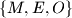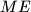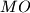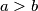MEMO 2012 ekipno problem 3

Kvaliteta:
Avg: 0.0
Težina:
Avg: 0.0
Let$n$ be a positive integer. Consider words of length$n$ composed of letters from the set$\{ M, E, O \}$. Let$a$ be the number of such words containing an even number (possibly 0) of blocks$ME$ and an even number (possibly 0) blocks of$MO$ . Similarly let$b$ the number of such words containing an odd number of blocks$ME$ and an odd number of blocks$MO$. Prove that$a>b$.
Source: Srednjoeuropska matematička olimpijada 2012, ekipno natjecanje, problem 3